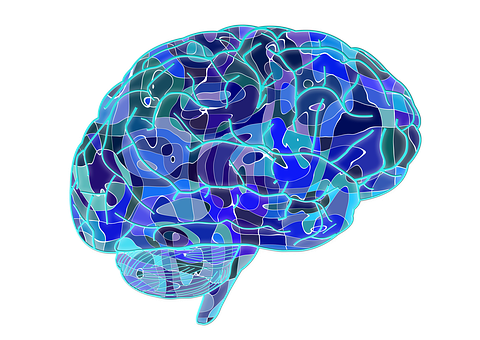# Chemistry 20 Most important Mcqs(1) Who predicted that all particles have wave-like properties.

(a) Einstein

(b) Schrodinger

(c) Bohr

(d) Broglie

(2) The force of attraction or repulsion between two charges is determined by.

(a) Newtons law

(b) Einsteins law

(c) Coulombs law

(d) None of these

(3) The first important discovery about the nature of cathode rays is made by.

(a) J.Perrin

(b) De-Broglie

(c) Crooks

(d) Hittorf

(4) Crooks in 1879, demonstrated that the cathode rays are actually streams of particles which have.

(a) Momentum

(b) Kinetic energy

(c) Both momentum and kinetic energy

(d) None of these

(5) Cathode rays possess.

(a) Negative charge

(b) Positive charge

(c) Neutral

(d) None of these

(6) The wavelength of green light lies in the range of.

(a) 510-580nm

(b) 400-450nm

(c)300-400nm

(d) 600-700nm

(7) Who proposed that electron in any atom may be considered as standing waves.

(a) Einstein

(b) Germer

(c) Heisenberg

(d) E.Schrodinger

(8) According to Heisenberg uncertainty principle, we cannot measure.

(a) Position

(b) Momentum

(c) Energy

(d) Position and Momentum

(9) Quantum mechanical model   of atom developed on the basis of Schrodinger equation helps to calculate.

(a) Bond energy

(b) Bond lengths

(c) Shapes of molecules.

(d) All of the above

(10) Electron behaves like.

(a) Wave

(b) particle

(c) Wave and particle

(d) None of these

(11) SI unit of wave length is.

(a) Meter cube

(b) Meter

(c) Meter square

(d) per meter

(12) The activity of radio isotope changes with

(a) Temperature

(b) Pressure

(c) Chemical environment

(d) None of the above

(13) In nuclear reactors the speed of Neutrons  slowed down by.

(a) H2O

(b) Zn-rods

(c) Uranium-rods

(d) D2O

(14) Schmidt observed invisible radiation in.

(a) Thorium

(b) Tellurium

(c) Titanium

(d) Technicium

(15) The equivalence of mass and energy was stated mathematically by.

(a) Einstein

(b) Bohr

(c) Oppenheimer

(d) Curie

(16) Which of the experiments convince us about the divisibility of atom.

(c) Rutherford experiment

(c) All of the above

(17) Alpha particles are.

(a) Neutrons

(b) Protons

(c) Electrons

(d) Helium nuclei

(18) The spontaneous emission of radiation from a radioactive substance is called.

(b) Fusion

(c) Fission

(d) Evaporation

(19) The beam attracted towards the negatively charged plate are.

(a) Alpha particles

(b) Neutron

(c) Beta particles

(d) Gamma rays

(20) Beta-rays are fast moving.

(a) Neutrons

(b) Positrons

(c) Electrons

(d) Deuteron